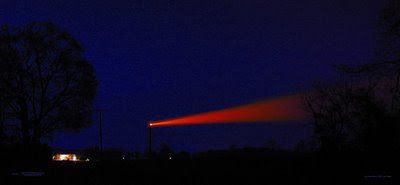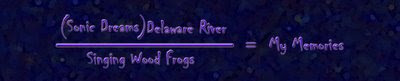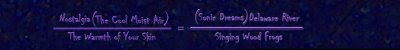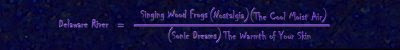Wednesday, July 09, 2008

Substitution in Mathematical PoetrySubstitution in Mathematical Poetry
This Blog entry will show an example of substitution in mathematical poetry. Substitution can occur when we have two equations that have a common term. For example let’s look at the two equations which have the same form as two similar triangles poems: A = BD/E and A = HJ/U since both equations have the term A’ in common and consequentially they both happen to be solved for ‘Athen we can set both equations equal to each other as such:
BD/E = HJ/U
We know that we can solve for any of the variables in our new equation and get a new equation in terms of one variable. Let do so and solve for J so we now have: J=UBD/EH
So now let’s apply what we have just witnessed to two similar triangles poems.
First of all we must look at the following two poems.We know from our earlier example that we can solve a mathematical equation for any term in it. If we take the first poem and solve it for “my memories” we then can present the poem as:Notice (below) that we have the two poems solved for the same term (my memories).Now we can set each poem equal to each other because they both have identical terms. (see below)We also know that we can solve this poetic equation for any of the terms in it. So let us solve this poem in terms of “Delaware River”Now we can see that the later poem was derived from the two similar triangles poems shown at the top. What is interesting is that all of the logical processes used to create the first two poems are contained in our resultant poem including the subtle differences in the contexts of each initial poem.
Substitution can also be used in poems created by different poets as long as they have a common term. Follow this link to collaborative substitution poems.

The following polyaesthetic piece uses the image of a shipping beacon located at Cedar Swamp on the Delaware side of the Delaware River. The full Delaware River Poem from our example is nestled in the lower left hand corner of the image. The physical size of the digital image is 67” x 31”